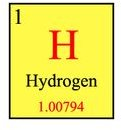# Answers to - How Do I Calculate Atomic Mass?

Page content

“How do I calculate atomic mass?” is a very common question for beginning chemistry students. The definition of atomic mass is the approximate sum of protons and neutrons in an atom’s nucleus. The electrons outside the nucleus can be ignored as their mass is so tiny as to be negligible. Atomic mass numbers for all known elements are listed on the periodic table.

Atomic mass and atomic weight are terms used interchangeably. However, mass is the amount of matter in an object and weight is the amount of mass times the gravitational pull on an object which makes atomic mass the preferred term.

## Equation

Scientists refer to the mass of atoms in atomic mass units or amu. An atomic mass unit is equal to 1/12 the mass of a carbon -12 atom. The atomic mass of an atom is the number of times it is heavier than 1/12 an atom of C-12. The atomic mass of hydrogen is 1 amu; an atom of hydrogen is 1/12 the mass of an atom of C-12.

Grams are the standard unit to express mass but because atoms are so small, grams or milligrams are not convenient to express the numbers of atomic mass. For example, the mass of a single atom of oxygen-16 would be 0.000,000,000,000,000,000,000,026,57 grams. Using such small numbers in calculations would be too complicated.

.

## Mass NumbersThe average atomic masses of all known elements are listed on the periodic table. These are used to calculate the atomic mass of compounds.

You may wonder why these numbers are usually not whole numbers. For example, hydrogen has an atomic mass of 1.00794. The reason for this is that most elements consist of two or more isotopes. Isotopes are forms of an element with the same atomic number (same number of protons) but different atomic masses due to different numbers of neutrons.

The mass number shown on the periodic table is an average of the mass of the isotopes of the element taking into account the abundance of the isotope’s occurrence in nature.

To calculate the atomic mass of a compound you will need a calculator, periodic table, pencil and paper.

1. Write out the chemical formula of the compound. For example: H2O.

2. Next, check the periodic table for the atomic mass of the elements used. Example: Hydrogen = 1.0079, Oxygen= 15.9994.

3. Next multiply the number of atoms of each element by the atomic mass of the element. Example: You have two hydrogen atoms times the mass. 2 x 1.0079= 2.0158. There is only one oxygen atom: 15.9994.

4. Finally, add the multiplication results. 2.0158 + 15.9994 = 18.0098. The atomic mass of the compound H2O is 18.0098.

Atomic mass is a basic chemistry concept. Defining and calculating atomic mass is not difficult; you just need the correct tools and an understanding of the chemistry terms.

Image Credits: Wikimedia Commons/The Sun. Wikimedia Commons/Booyabazooka

Sources:

https://www.scienceclarified.com

https://www.tutorvista.com/science/

https://www.nuce.boun.edu.tr/glossary.html